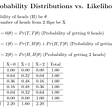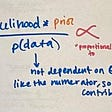Budget and Budget Set

# What is budget?

Budget is a financial plan for a defined year which is based on the income of an individual.

for E.G- if the person wants to buy a car but his annual income is not much then he or she will think twice before buying.

# What is Budget Set?

BUDGET SET- THE collection of all bundles of goods that consumers can buy with her income at existing market price. to understand it clearly lets take an example……..

The income of the person be M and prices of apples and mangoes be P1 and P2 and let the quantity which consumer wants to buy be X1 and X2.

So if the person wants to buy apples then he or she have to spend equal amounts of money that is P1. X1.

Similarly ,if the person wants to buy mangoes then he or she have to spend equal amount of money that is P2.X2.

Suppose if the person wants to buy the bundle consisting X1 amount of apples and X2 amounts of mangoes. Then he or she will have to spend P1.X1+P2.X2 amount of money.

Example forthe first equation- let the amount of the apples be i.e- P1= 10 for a piece. The consumer wants to buy around 10 pieces then it will be like this-:

P1=10*X1=10

10*10=100, so the consumer will spend 100 rupees for the apples.

Now example for the second equation- let price of the mangoes be i.e P2=20 for the piece. The consumer wants to buy around 8 pieces then it will be like this-:

P2=20*X2=8

20*8=160,so the consumer will spend 160 rupees for mangoes.

Now for the third equation the case is bit different. So lets just assume that there are 20 pieces of each in the bundles.

So now according to the third equation, consumer wants to buy bundles of each goods. Now it will be like this.

P1.X1+P2.X2

Now as we know the rates of apples and mangoes are 10 and 20 respectively and let’s assume that there are 20 pieces in one bundle and the consumer wants to buy two bundles that is one of apples and other is of mangoes.

Then,

By putting the equation written above-:

P1=10 and X1=20

Similarly, P2=20 and X2=20

As we know P1.X1+P2.X2 will be the total amount of the third equations.

P1.X1=10*20

P2*X2=20*20

So it will be like this ,

10*20=200

20*20=400

10*20+20*20=600 so the consumer will pay 600 for both of the bundles.

But there is a condition that consumer can buy only the bundles as long as it costs less than or equal to his or her income.( in other words consumer can buy any bundle such that-:

P1.X1 +P2.X2 ≤ M , where M is the budget or money he has with himself or salary

The above equation is what we call the Budget Constraint.

And whatever values satisfies the above equation will form the budget set for the individual.

Assuming, the person’s budget is 500, we can say the above purchase of 8 mangoes and 10 apples which amounted to 160 +100 = 260 rupees satisfies the above condition, therefore it is a part of the budget set for the individual.

*(.) symbolizes multiplication

--

--

--

## IMO 2021 — Results and Analysis## An Olympiad Combinatorics Problem with a Beautiful Geometric Solution## Game Theory## Maximum Likelihood Estimation VS Maximum A Posterior## Math news: Reporters## Equivalence (Likelihoods)## Flat Priors## How to never work in life again by creating your own passion?## Learn Timeless Life Lessons from the Great Books## 6 Ways AI Can Increase Donor Funding